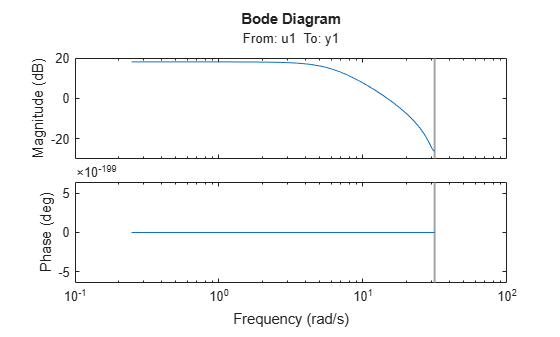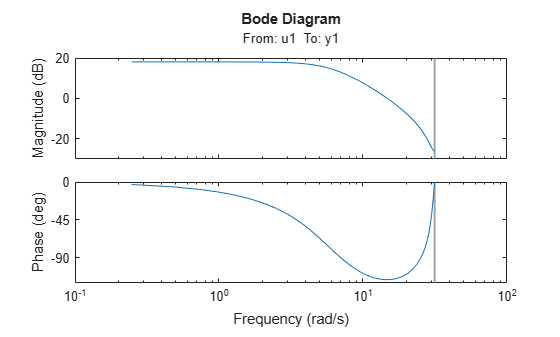Add minimum phase to frequency response magnitude

## Syntax

``datac = addMinPhase(data)``
``datac = addMinPhase(data,ts)``

## Description

````datac = addMinPhase(data)` adds phase information to the magnitude-only frequency-response signals stored in `data`.Use `addMinPhase` to generate phase information for any complex vector H(w) for which the measured magnitude |H| is available but not the phase. `addMinPhase` assumes that H(w) represents the frequency response of a minimum-phase stable linear system.When `data` is numeric, the sample time is assumed to be one second and the frequency is assumed to be `linspace(0,pi,size(data,1))`.```

example

````datac = addMinPhase(data,ts)` replaces the default sample time with the sample time specified in `ts`. Units are in seconds.```

## Examples

collapse all

Load time-domain input-output data and store the frequency response in an `idfrd` object. Remove the phase information by taking the absolute value of the response. Plot the response.

```load iddata1 z1 m0 = arx(z1,[2 3 0]); ts = z1.Ts; w = linspace(0,pi,128)/ts; response = idfrd(m0,w); response.ResponseData = abs(response.ResponseData); bode(response)```Add the minimum phase information to the response. Plot the response.

```responseMinPhase = addMinPhase(response,ts); bode(responseMinPhase)```## Input Arguments

collapse all

Input frequency response signals, specified as one of these values:

• A matrix of positive real values

• An `idfrd` object containing positive real values in its `ResponseData` property.

Sample time of signals, in seconds, specified as a nonnegative real scalar.

## Output Arguments

collapse all

Frequency response signals with minimum phase added, returned as a complex matrix or as an `idfrd` object.

• When `data` is a matrix, then `datac` is a complex matrix of the same size as `data`. If the vector `data(:,i)` has all real and nonnegative values, then `datac(:,i)` is the complex version of `data(:,i)` with added phase. Otherwise, `datac(:,i)` is identical to `data(:,i)`.

• When `data` is an `idfrd` object, then `datac` is also an `idfrd` object. If the matrix `data.ResponseData(i,j,:)` has real and nonnegative values for each input-output pair `(i,j)`, then `datac.ResponseData(i,j,:)` is the complex version of that vector with added phase. Otherwise, `datac.ResponseData(i,j,:)` is identical to `data.ResponseData(i,j,:)`.

## Version History

Introduced in R2022b